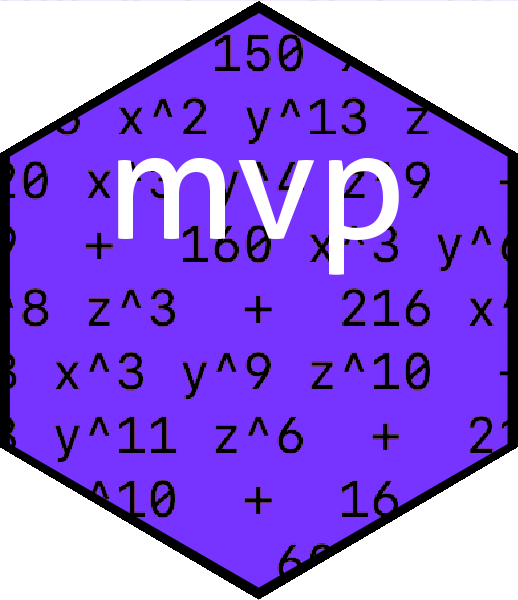# OverviewMultivariate polynomials are intereresting and useful objects. Here I present the `mvp` package which hopefully improves upon previous R functionality provided by the packages `multipol`, `mpoly`, and `spray`. The `mvp` package follows `mpoly` in using a symbolic, rather than numeric, representation of a multivariate polynomial; but it offers speed advantages over `mpoly`. `mvp` uses the excellent print and coercion methods of the `mpoly` package. `mvp` includes some pleasing substitution idiom not found elsewhere; it is theoretically comparable in speed to the `spray` package and I present some timings in the package vignette.

The `mvp` package uses `C++`’s STL `map` class for efficiency, which has the downside that the order of the terms, and the order of the symbols within each term, is undefined. This does not matter as the mathematical value of a multivariate polynomial is unaffected by reordering; and the print method (taken from `mpoly`) does a good job in producing human-readable output.

# Installation

You can install the released version of `mvp` from CRAN with:

``````# install.packages("mvp")  # uncomment this to install the package
library("mvp")``````

# The `mvp` package in use

Creating a multivariate polynomial is straightforward:

``````X <- as.mvp("1 + a^2 + a*b*c^3")
X
#> mvp object algebraically equal to
#> 1 + a b c^3 + a^2``````

and arithmetic operations work as expected:

``````Y <- as.mvp("12*a^2  + b - c^2 + 4*d")
X+Y
#> mvp object algebraically equal to
#> 1 + a b c^3 + 13 a^2 + b - c^2 + 4 d
X-3*Y
#> mvp object algebraically equal to
#> 1 + a b c^3 - 35 a^2 - 3 b + 3 c^2 - 12 d
X^2
#> mvp object algebraically equal to
#> 1 + 2 a b c^3 + 2 a^2 + a^2 b^2 c^6 + 2 a^3 b c^3 + a^4``````

Substitution uses the `subs()` function:

``````X
#> mvp object algebraically equal to
#> 1 + a b c^3 + a^2
subs(X,a=1)
#> mvp object algebraically equal to
#> 2 + b c^3
subs(X,a=1,b=2)
#> mvp object algebraically equal to
#> 2 + 2 c^3
subs(X,a=1,b=2,c=3)
#>  56
subs(X+Y,a="1+x^2",b="x+y",c=0)
#> mvp object algebraically equal to
#> 14 + 4 d + x + 26 x^2 + 13 x^4 + y``````

# Further information

For more detail, see the package vignette

`vignette("mvp")`